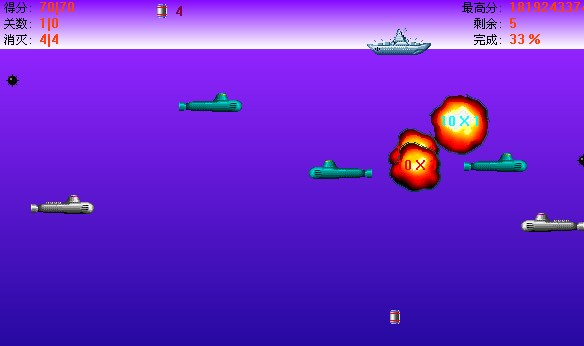# 2608. Battle of Submarines

A ship is on a W wide sea area. N enemy submarines numbered from 1 to N are underwater moving from west to east at

depth of D. Every two seconds the submarine can move one unit distance. The ship is equipped with unlimited bombs.

At each second, the ship can do one of the following actions:

1. Move one unit.

2. Release a bomb, and destroy the first submarine vertically under the ship.

3. Do nothing.At first, the ship’s moving direction is from west to east. It changes its moving direction if and only if the bomb destroys a

special type of submarine.

At no time can submarines overlap at each other.

Every submarine has an important degree. Given the time each submarine enters the sea area at the most west side, and

whether the submarine is the special type, please calculate the maximum sum of destroyed submarines’ important degree in

time [0, S] inclusive.

### 输入格式

The input consists of multiple test cases. The first line of input contains an integer T, which is the number of test cases.

The input consists of several test cases. Each test case starts with a line containing four integers W, D, N, and S. Each of

the following N lines contains four integers: Gi, Si, Di, Ci. Gi indicates the important degree of i-th submarine; Si is the

time the i-th enters the sea area at the most west side; Di is the submarine’s depth. If Ci is 1 then the submarine is the

special type, otherwise it is a normal one.

[Technical Specification]

T is an integer, and T <= 50.

W, D are integers, and 1 <= W, D <= 3.

N is an integer, and 1 <= N <= 30.

S is an integer, and 1 <= S <= 30.

Gi is an integer, and -1000 <= Gi <= 1000.

Si is an integer, and 0 <= Si <= S,

Di is an integer, and 1 <= Di <= D.

Ci is an integer, and Ci = 0 or 1.

### 输出格式

For each test case, print a single line containing a number indicating the maximum sum.

### 样例

Input
2
3 3 4 3
10 1 1 0
10 1 2 0
10 1 3 0
50 3 3 0
3 3 2 3
10 3 1 0
-10 0 1 0

Output
70
10


0 人解决，3 人已尝试。

0 份提交通过，共有 3 份提交。

9.9 EMB 奖励。